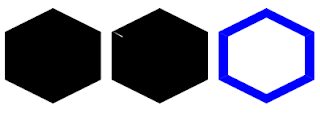## Thursday, September 15, 2011

### DDTe3 Hours 11 Drawing Hexes

In order to manipulate hex-shaped images, masks are going to have to be used. In canvas parlance, clipping regions are the proper term. Clipping is one of the ugliest parts of the canvas API, as it uses a shrinking region logic. You have to save the canvas state before setting up the region, set up the region, do the clipping based drawing, then restore the state of the canvas.  Thankfully clipping is powerful enough to allow paths to be used to create a clipping region.  This means that if we can create a simple hex-drawing function, that hex can be used as a clipping shape for creating hex-shaped puzzle pieces.

Actually, instead of drawing a hex, creating an array of points is more useful, especially when it comes time to creating the border. The hex, when you look at it, really only has a small number of important numbers that need to be calculated. Figure 1 shows the special points that are needed to create the hex and listing 1 shows the function used for creating the array of points.figure 1

function buildHexPath(rect, pathlist)
{
var path = pathlist;
// make sure path list of points contains proper number of points
if (path == null)
path = new Array();
while (path.length < 7)
path.push(new BGLayers.Point());
while (path.length > 7)
path.pop();

var halfx = rect.x + rect.width / 2;
var x2 = rect.x + rect.width;
var y1a = rect.y + rect.height / 4;
var y1b = rect.y + 3 * rect.height / 4;
var y2 = rect.y + rect.height;

path.x = rect.x;
path.y = y1a;
path.x = halfx;
path.y = rect.y;
path.x = x2;
path.y = y1a;
path.x = x2;
path.y = y1b;
path.x = halfx;
path.y = y2;
path.x = rect.x;
path.y = y1b;
path.x = rect.x;
path.y = y1a;
return path;
}

The hex can now be drawn, but to create a border we need a pair of hexes. My first attempt to create a border hex was to create an inner hex and an outer hex and join the two together. This did not work as expected. If you look at figure 2, the first hex is the normal hex. The second hex is suppose to be the border. My immediate reaction was that the fill method didn’t support complex polygons. This isn’t that surprising of a limitation so I quickly wrote my plan B implementation.figure 2

The third hex in this figure was created by drawing each segment of the hex separately. This was done in a similar way to the first attempt at creating a border. A outer hex and an inner hex are created and then the points are looped through and combined to create six separate polygons.

function drawHexBorder(ctx, rect, pct)
{
var outpath = buildHexPath(rect, null);
var wadj = rect.width * pct;
var hadj = rect.height * pct;
for(var cntr = 0; cntr < 6; ++cntr) {
ctx.beginPath();
ctx.moveTo(outpath[cntr].x,outpath[cntr].y);
ctx.lineTo(outpath[cntr+1].x,outpath[cntr+1].y);
ctx.lineTo(inpath[cntr+1].x,inpath[cntr+1].y);
ctx.lineTo(inpath[cntr].x,inpath[cntr].y);
ctx.lineTo(outpath[cntr].x,outpath[cntr].y);
ctx.closePath();
ctx.fill();
}
}

Once this was done I decided to spend a little bit of time researching how the cavas fill was suppose to work as I was positive that I had seen some drawings that had holes in it. After a few minutes of research (about the same amount of time it took me to write plan-b) I finally stumbled upon the winding rule for filling. It appears that the direction of the edge lines are used as part of determining of the points that are filled. While I have played with a number of fill algorithms, I have never actually implemented a winding algorithm but it does mean that by reversing the order of the points in the inner hexagon, the fill should work properly. This would allow the drawing of the border as a single drawing operation which should be more efficient.